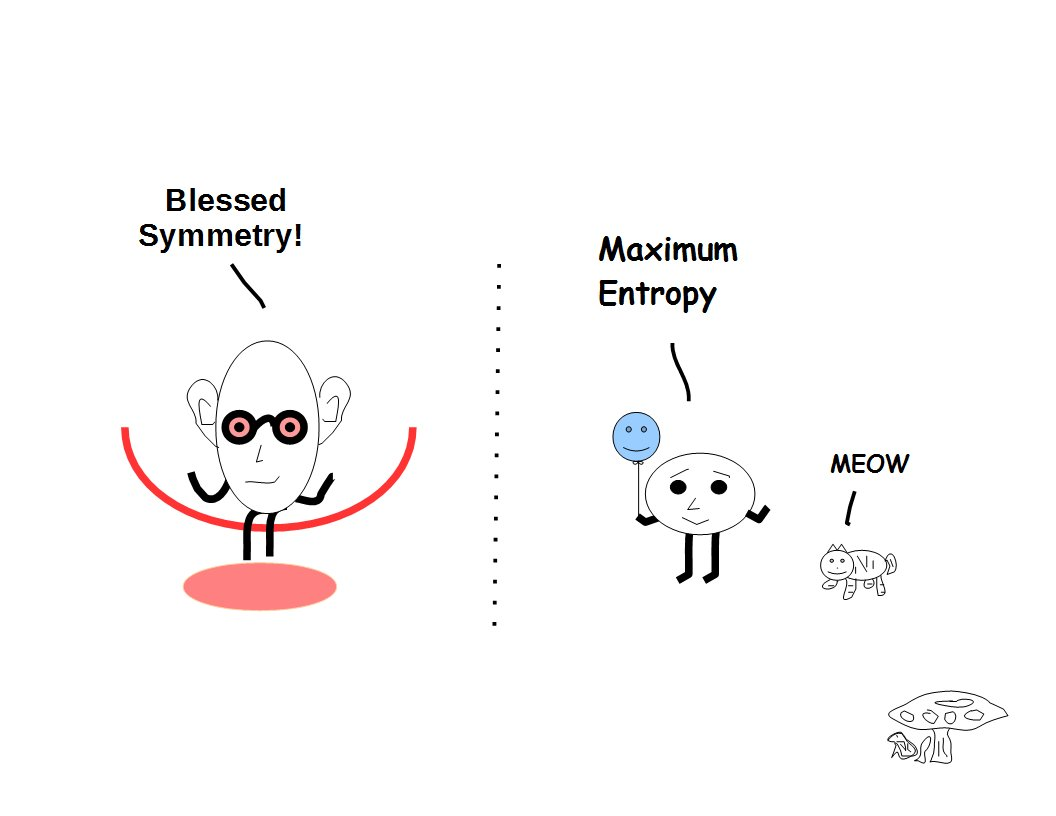# ijspace.org

 Set Theory in j-space 1st March 2015           The fundamental concepts in physics or science in general require a certain logical structure based on mathematics, while mathematics itself traces its roots deep in to the philosophical reasoning. The set-theory based on Zermelo-Fraenkel Axioms, is one such example. The basic definition of Set allows membership of objects distinct from each other. Therefore it is an obvious choice to represent the j-space. When we consider j-space where every member represents a measurements, it becomes a very interesting exercise to evaluate set-theory axioms. Before we launch into developing a description in physical space of a macroscopic observer, it is worthwhile to understand what these fundamental axioms are trying to tell us. Predictably we will have to transition over to topological space, once we start discussing the concept of continuity in a discrete measurement space.     We have to keep in mind that to define a Set of measured objects, we have to define the observer metric under which the measurements are being made. The metric is based on the observer's capacity. The observer's capacity translates into the definition of the origin which itself is guided by the VTS symmetry. Hence to define a Set we need to define "Quanta" and the extent of our measurement space. Important to note is that the definition of quanta will vary per individual observer's capacity and so will be the definitions of "Null", "Zero", and "Origin". We are stating "Origin" explicitly as it includes the geometrical concept of convergence. We also know that a discrete space can not be source free and it has to have the rotational property per Obsi criterion, even though the macroscopic observer may measure it otherwise. Both of these properties are ingrained in any set formed in j-space. For discussion purposes Quanta, Source and Rotational properties will be assumed to be implicit to the description as measured by a given observer. We will mention them only when descriptions measured by the observers of different capacities are compared.        How about subsets? Consider a set {1, 2, 3}. Some of the subsets are {1}, {1, 2}, and {1, 2, 3}. So how to understand these subsets where some measurements, e.g. 1 or 2, are repeated to form individual subsets. Each of them represent an observation, and 1, 2, and 3 have been measured only once and they should be unique. So in order form subsets {1} and {1, 2} for example, we will have to measure 1 twice and 2 at least once.  Similarly to form subsets {1}, {1, 2}, and {1, 2, 3}, we will have to measure 1 three times, 2 twice and 3 at least once. The information represented by each measurement of "1" will be slightly different from each other, although we can assume that the difference will be below observer's measurement threshold. Maybe we can think of symmetries which allow us to visualize clusters of 1's and 2's and so on. As a consequence the members of any set in j-space are likely to share some type of symmetry property common to them, if we wish to form subsets or power-sets. (For example, we know that rather than the same electron going back and forth in time, all electrons are different from each other in j-space even though we measure them alike.)     Similar considerations can also be made for the arithmetic operations such as addition, subtraction, and multiplication. For example in the relationship 1 + 1 = 2, 2 is the result of the addition performed on two supposedly identical PE1 measurements represented as 1 and 1. But in an entropic space it is not possible to perform two identical measurements, unless we assume them identical using some symmetry or in other words the strict PE=1 definition modifies to more flexible PE~1 . We have intentionally not included the operation "ratio" in this category.     If we think about it, in j-space the number system is not possible without defining quanta or VTS or mathematically "0". We have to understand that per Obsi the measurement of any "0" defined by any macroscopic observer, will always be finite "0j". We can appreciate that the information structure for a given set will change with change in the definition of "0j". Similarly the description of the measurement space for each macroscopic observer, will have different significance for the numbers. For example our friend Zörn's definition of 1q=2 will have much more information than Joe's definition of 1q=3.     We can also think of the significance of prime numbers in j-space. A prime number has to be in Natural number space, but then in the measurement space what is the definition of a natural number? A measurement considered absolute or PE1, by a macroscopic observer essentially is a prime measurement, but Obsi will never agree to it. Thus prime numbers do have significance, but only within the measurement space defined by the macroscopic observer using VTS. Similarly we may want to think about the meaning of the continuity and the role real numbers play in defining it.       It will be prudent to clarify that the quantum nature in j-space is due to the limited capacity of the observer making the measurements. A measurement is not possible without interactions in j-space. In fact j-space is an interaction space and therefore a measurement space. We will discuss ZFC axioms in j-space next. (Oh, by the way, why would the capacity of an observer be of quantum nature to begin with?)   To be continued...Information on www.ijspace.org is licensed under a Creative Commons Attribution 4.0 International License.